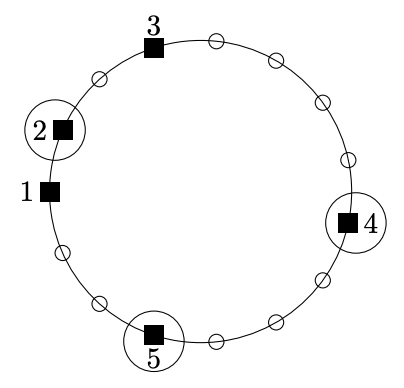시간 제한 메모리 제한 제출 정답 맞은 사람 정답 비율
1.5 초 64 MB 75 14 14 31.111%

## 문제

Johnny went camping together with his friends. They want to use the huts at the camping ground, which are all located on a circle, not necessarily at the same distances (for simplicity, we will measure the distance along the circle). Each member of the group, including Johnny, may choose any hut--there are at least as many huts as friends in the group. However, everybody wants some privacy and it is desirable that the distances between occupied huts are large; clearly each hut is meant for a single occupancy. Help them in planning their holidays: write a program that will determine the set of huts to be occupied, so that the minimal distance (measured along the circle) between them is maximal possible.

## 입력

First line of the input contains two positive integers $n$ and $k$ ($2 \le k \le n \le 500\,000$), denoting: the number of huts and friends (including Johnny). The second and last line contains a sequence of $n$ positive integers $a_i$ ($1 \le a_i \le 10^9$), denoting the distance between the hut number $i$ from the hut number $i+1$ (for $i < n$) or the distance between hut $n$ and $1$ (for $i = n$). All distances are measured along the circle.

## 출력

You should write a single positive integer: the maximal, among all sets of $k$ occupied huts, minimal distance between two occupied huts. Again, the distance is measured along the circle.

## 예제 입력 1

5 3
1 2 5 4 3


## 예제 출력 1

4


## 힌트

Situation looks like on picture below:Friends can choose huts numbered $2$, $4$ and $5$. Then distances between neighbouring selected huts are: $7$, $4$ and $4$.